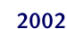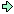World!Of
NumbersWON plate
122 |

[ January 9, 2002 ]
Numbers equal to the sum of (at least two) consecutive integers
& numbers equal to the sum of (at least two) consecutive primes

As a consequence of findingequal to
the sum of consecutive positive integers in seven ways - notation (#,F)
Let # = number of consecutive integers
Let F = first integer of the sequence

then 2002 equals
(4,499) = (7,283) = (11,177) = (13,148) = (28,58) = (44,24) = (52,13)
I asked Carlos Rivera and Terry Trotter if there exist
a mathematical procedure to find all combinations.
Is there a way to get them all straightaway,
not only for 2002 but for any arbitrary integer ?Carlos replied with
No more integer solutions other than the seven found, for b in
S=(a+b)(b-a+1)/2 = 2002, for 1 < = a <= 1001
ps. a is the first member of the sequence
and b is the last member of the sequence.Terry used his Excel spreadsheet to solve
the problem in a more elaborate way.
The basic equation is
nx + n(n – 1)/2 = 2002
and he set it up this way

 cell A1 = 1 B1 is empty C1 is empty cell A2 = A1 + 1 B2 = A2*(A2–1)/2 C2 = (2002-B2)/A2 cell A3 = "copy" of A2 B3 = "copy" of B2 C3 = "copy" of C2 ... ... ... cell A63 = "copy" of A2 B63 = "copy" of B2 C63 = "copy" of C2

For any arbitrary integer, replace 2002 with another number
E.g. 2001 gives integer solutions for

3 terms, starting with 666
6 terms, starting with 331
23 terms, starting with 76
29 terms, starting with 56
46 terms, starting with 21
58 terms, starting with 6

now, for next year, 2003 gives only

2 terms, starting with 1001

Terry excelled further and got more data regarding
sums of consecutive positive integers

2000 has 3 ways(5,398) (25,68) (32,47)
2001 has 6 ways(3,666) (6,331) (23,76) (29,55) (46,21) (58,6)
2002 has 7 ways(4,499) (7,283) (11,177) (13,148) (28,58) (44,24) (52,13)
2003 has 1 way(2,1001)
2004 has 3 ways(3,667) (8,247) (24,72)
2005 has 3 ways(2,1002) (5,399) (10,196)
2006 has 3 ways(4,500) (17,110) (59,5)
2007 has 5 ways(2,1003) (3,668) (6,332) (9,219) (18,103)
2008 has 1 way(16,118)
2009 has 5 ways(2,1004) (7,284) (14,137) (41,29) (49,17)
2010 has 7 ways(3,669) (4,501) (5,400) (12,162) (15,127) (20,91) (60,4)Terry worked a little more and allowed negatives as well as positives.
The following new items emerged
2002 has 3 more ways(#77,–12, up to 64) (#91,–23, up to 67) (#143,–57, up to 85)
All can be verified by students as they use the famous
textbook formula S = (n/2)[2a + (n–1)d]The analogue case for numbers as
sums of (at least two) consecutive positive primes
seems much more difficult !

Some examples for 2002
491 + 499 + 503 + 509 (#4)
107 up to 179 (#14)

Carlos Rivera formulated a hard but nice challenge
Who can provide the following sequence ?
The earliest numbers that are expressible as a sum of consecutive primes
in K ways, for K = 1, 2, 3, ... 20.

The earliest primes expressible as...
were already covered by Carlos (cr) in his own primepuzzle website
yet he gave also some solutions for the smallest nonprimes.
See cases K = 6 and K = 7.

 K Earliestnumber Sums of (at least two)consecutive primes Who Earliestprime 1 5 (#2,2)2 + 3 pdg 5 2 36 (#2,17)17 + 19 (#4,5)5 + 7 + 11 + 13 pdg 41 3 240 (#2,113) (#4,53) (#8,17) pdg 311 4 311 (#3,101) (#5,53) (#7,31) (#11,11) pdg 311 5 16277 (#7,2297) (#11,1451) (#13,1213)(#35,359) (#37,331) pdg 34421 6 130638 (#8,16273) (#10,13009)(#12,10847) (#56,2113)(#140,461) (#206,29) cr 442019 7 218918 (#12,18199) (#16,13619)(#22,9851) (#28,7691)(#38,5623) (#46,4561)(#62,3301) cr 3634531 8 ? ? ? 48205429 9 ? 10 ? 11 ? 12 ? 13 ? 14 ? 15 ? 16 ? 17 ? 18 ? 19 ? 20 ?A000122Prime Curios!Prime PuzzleWikipedia 122Le nombre 122```

```

[TOP OF PAGE]

Patrick De Geest - Belgium- Short Bio - Some Pictures